# Plus Two Physics Chapter Wise Questions and Answers Chapter 15 Communication Systems

Students can Download Chapter 15 Communication Systems Questions and Answers, Plus Two Physics Chapter Wise Questions and Answers helps you to revise the complete Kerala State Syllabus and score more marks in your examinations.

## Kerala Plus Two Physics Chapter Wise Questions and Answers Chapter 15 Communication Systems

### Plus Two Physics Communication Systems NCERT Text Book Questions and Answers

Question 1.
Frequencies in the UHF range normally propagate by means of:
(a) ground waves
(b) sky waves
(c) surface waves
(d) space waves
(d) space waves.Question 2.
Digital signals
(i) do not provide a continuous set of values
(ii) represent values as discrete steps,
(iii) can utilize only binary system, and
(iv) can utilize decimal as well as binary system. Which of the following options is True:

(a) Only (i) and (ii)
(b) Only (ii) and (iii)
(c) Only (i), (ii) and (iii) but not (iv)
(d) All the above (i) to (iv)
(c) because decimal system implies continuous set of values.

Question 3.
Is it necessary for a transmitting antenna to be at the same height as that of the receiving antenna for line of sight communication? A T.V. transmitting antenna is 81 m tall. How much service area it can cover if the receiving antenna is at the ground level.
No.Question 4.
A modulating signal is a square wave as shown below: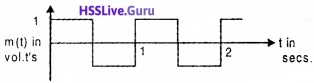The carrier wave is given by c (t) = 2 sing (8πt) volts.

1. Sketch the amplitude modulation wave form.
2. What is the modulation index?

1. amplitude modulation wave form is as shown below: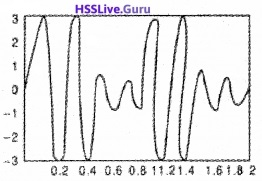2. µ = 0.5

### Plus Two Physics Communication Systems One Mark Questions and Answers

Question 1.
For base station to mobile communication, the required frequency band is
(a) 540 – 1600 kHz
(b) 200 – 325 MHz
(c) 5.9 – 6.42 GHz
(d) 840 – 935 MHz
(d) 840 – 935 MHz.Question 2.
What do you mean by modulation index in AM?
It is the ratio of the change in amplitude of the modulated carrier wave to the amplitude of the original carrier wave.

Question 3.
A communication between a fixed base station and several mobile units, located on ships or aircraft utilizing two way radio communication in the VHF and UHF is of frequency band
(a) 3 to 30 MHz
(b) 30 to 300 MHz
(c) 30 to 470 MHz
(d) 30 to 600 MHz
(c) 30 to 470 MHz.Question 4.
Which of the following frequency/frequencies will be suitable for beyond the horizon communication using sky waves?
(a) 10 kHz
(b) 10 MHz
(c) 1 GHz
(d) 1000 GHz
(b) 10 MHz.

Question 5.
What are the different types of message signals used in a communication system?
The different type of message signals are voice, music, picture and computer data.Question 6.
What do you mean by modulation index in AM?
It is the ratio of the change in amplitude of the modulated carrier wave to the amplitude of the original carrier wave.

### Plus Two Physics Communication Systems Two Mark Questions and Answers

Question 1.
Arrange the contents in the table in the most appropriate way.

 A B Low frequency ratio waves Space wave propagation Television transmission Ground wave propagation High frequency radio wave Geo stationary satellite Microwaves Sky wave propagation

 A B Low frequency ratio waves Ground wave propagation Television transmission Space wave propagation High frequency radio wave Sky wave propagation Microwaves Geo stationary satelliteQuestion 2.
What is the modulation index of AM, if the voltage amplitude of the carrier wave is 2kV and side band voltage amplitude is 200V.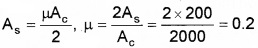Question 3.
100 Hz is an audio frequency. Its wave length is 3000 km. What is the minimum length of the antenna to transmit this wave?
C = νλ
3 × 108 = 100 × λ
λ = $$\frac{3 \times 10^{8}}{100}$$m = 3 × 106m
Length of antenna I = $$\frac{\lambda}{4}=\frac{3 \times 10^{6}}{4}$$ = 7.5 × 105m.

### Plus Two Physics Communication Systems Three Mark Questions and Answers

Question 1.
An expert said that it is necessary to modulate low frequency signals to sent long distances.

1. What is meant by modulation
2. What are the different types of modulation

1. Modulation is the process of super posing a low frequency (audio signal) information on to a high frequency carrier wave.

2. Three types of modulation using sinusoidal carrier waves

• Amplitude modulation
• Frequency modulation
• Phase modulationQuestion 2.
The type of communication system needed fora given signal depends on the band of frequencies in the signal

1. What are the different types of message signals used in a communication systems. (1)
2. Match the bandwidth given in Column B with the signal given in Column A.
 Column A Column B speech signals 6 MHz Music signals 2.8 kHz Video signals 20 kHz TV signal 4.2 MHz

1. The different type of message signals are voice, music, picture and computer data.

2.

 Column A Column B speech signals 2.8 kHz Music signals 20 kHz Video signals 4.2 MHz TV signal 6 MHz

### Plus Two Physics Communication Systems Four Mark Questions and Answers

Question 1.
The height of a transmitting antenna is 200m. Radius of earth is 6.4 × 106m
1. Which one of the following is used in TV transmission?

• Sky wave
• Space wave
• Groundwave
• None of these

2. Find the range upto which the above antenna gives transmission signal.

3. Why is it necessary to use satellite for long distance transmission?
1. Space wave

2.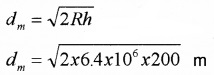3. Space waves can not be reflected back by iono sphere. Space wave can be reflected back to earth by making use of artificial satellite.Question 2.
A radio transmitter, microwave transmitter, and a microwave receivers are placed in a hilly area.

2. In this situation, what are your suggestions for proper communication? Are Microwaves suitable there?

1. Yes. Because wavelength of radio wave is larger than microwave.
2. To have large coverage, increase the height of antenna or stations. Microwaves are not suitable for this kind of transmission.

Question 3.
The given block diagram shows general form of a communication system.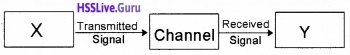1. Identify the parts X and Yin the diagram.
2. What is the difference between

• Attenuation and Amplification

3. Repeaters are used;

• To decrease the range of a communication system.
• To increase the range of a communication system
• Both (i) and (ii)

2. difference between:

• A transmitter converts message signal into a form suitable for transmission. A receiver receives the transmitted signal and reconstructs the message signal.
• The loss of strength of signal while passing through the medium is known as attenuation. The process of increasing the amplitude of a signal is known as amplification.

3. Repeaters are used to increase the range of a communication system.Question 4.
The given figure shows the various propagation modes of e.m. waves for communication.1. Identify the propagation modes in A, B, C
2. Why transmission of TV signals via sky wave is not possible?
3. A radio can tune to any station in 7.5 MHz. to 12 MHz. What is the corresponding wavelength band?

1. A – ground wave, B – space wave, and C – sky wave.

2. TV signals are in the range of 100 to 220MHz. Ionosphere cannot reflect these waves.

3. Wave length corresponding to 100 MHz is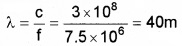Wavelength corresponding to 220 MHz is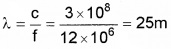the wavelength band is 25m to 40m.Question 5.
To transmit signals in the range 30 – 300 MHz space wave propagation is used

1. What is meant by space wave propagation?
2. Why it is called Line of Sight (LOS)?
3. Derive an expression for area covered by a sig¬nal if height of antenna is h

1. In space wave propagation, wave travels in straight line from transmitting antenna to the receiving antenna.
2. Because the receiving antenna and transmitting antenna should be in line of sight of wave.
3. Range of signal from the antenna d = $$\sqrt{2 \mathrm{Rh}}$$

∴ Area A = πd2 = π ($$\sqrt{2 \mathrm{Rh}}$$)2
A = π 2Rh.Question 6.
In the broadcast mode of communication, modulation is necessary.

1. What do you mean by modulation? (1)
2. Why modulation is necessary? (2)
3. What do you mean by modulation index in AM? (1)

1. Modulation is the process by which some characteristics, like amplitude, frequency or phase angle of a high frequency carrier wave is varied in accordance with the instantaneous value of the audio signal.

2. Modulation is necessary due to the following reasons.

• The height of the transmitting antenna should be at least 1/4th of the wave length. So to transmit audio frequency, we need very long antenna.
• The power radiated by an antenna is inversely proportional to the square of the wavelength. In audio frequency, wavelength is higher.

3. It is the ratio of the change in amplitude of the modulated carrier wave to the amplitude of the original carrier wave.Question 7.
The given figure shows the frequency spectrum of an amplitude-modulated wave.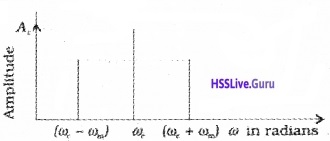1. What are the names of (ωc – ωm) and (ωc + ωm). (1)
2. What is the value of amplitude of (ωc – ωm) if modulation is µ? (1)
3. What is the modulation index of AM, if the voltage amplitude of the carrier wave is 2kV and side band voltage amplitude is 200V. (2)

2. Value of the amplitude of the side band frequency is As = $$\frac{\mu A_{c}}{2}$$ where Ac is amplitude of carrier.